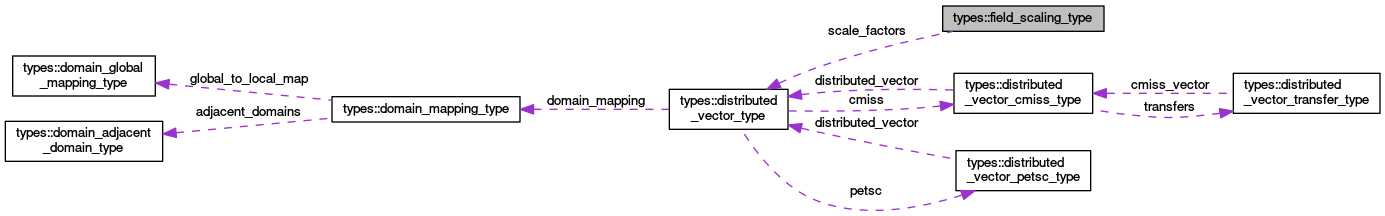OpenCMISS-Iron Internal API Documentation
types::field_scaling_type Type Reference

A type to hold the scale factors for the appropriate mesh component of a field. More...

Collaboration diagram for types::field_scaling_type:[legend]

## Public Attributes

integer(intg) mesh_component_number
The mesh component number of a field variable component that the scaling factors are associated with. More...

integer(intg) max_number_of_derivatives
The maximum number of derivatives in the mesh component. More...

integer(intg) max_number_of_element_parameters
The maximum number of element parameters in the mesh component. More...

type(distributed_vector_type), pointer scale_factors
SCALE_FACTORS(nk,np). The scale factor that is applied to the nk'th derivative of the np'th node of the mesh component. More...

## Detailed Description

A type to hold the scale factors for the appropriate mesh component of a field.

Definition at line 1168 of file types.f90.

## Member Data Documentation

 integer(intg) types::field_scaling_type::max_number_of_derivatives

The maximum number of derivatives in the mesh component.

Definition at line 1170 of file types.f90.

 integer(intg) types::field_scaling_type::max_number_of_element_parameters

The maximum number of element parameters in the mesh component.

Definition at line 1171 of file types.f90.

 integer(intg) types::field_scaling_type::mesh_component_number

The mesh component number of a field variable component that the scaling factors are associated with.

Definition at line 1169 of file types.f90.

 type(distributed_vector_type), pointer types::field_scaling_type::scale_factors

SCALE_FACTORS(nk,np). The scale factor that is applied to the nk'th derivative of the np'th node of the mesh component.

Todo:
Make scale factors nodally based for now. Will have to revert to element based and extended to be a matrix to allow for a global derivative to be mapped onto many different element derivatives at the points that closes meshes or for inconsistent xi directions

Definition at line 1172 of file types.f90.